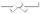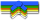# Exercises

0 / 50

Write a function in Java that implements the following logic: Given a string, return a string made of its first 2 chars. If the string...0 / 50

Write a function in Java that implements the following logic: Given a string, return a string of length 1 from its front, unless `front` is...0 / 10

Write a function in Java called `factorial()` that will take a positive integer as input and returns its factorial as output.0 / 50

Write a function in Java that implements the following logic: Given a string and an int `n`, return a string made of `n` repetitions of the...0 / 50

Write a function in Java that implements the following logic: Given a string `str` and a non-empty `word`, return a version of the original...0 / 50

Write a function in Java that implements the following logic: Given two strings, `a` and `b`, create a bigger string made of the first...0 / 50

Given a string `str`, find all places where a three-letter combination starting with "z" and ending with "p" occurs. Return a string where...0 / 50

Write a function in Java that implements the following logic: Given a string `str` and a non-empty `word`, return a string made of each...0 / 50

Write a function in Java that counts the number of times the string "code" appears anywhere in the given string `str`, except that we'll...0 / 50

Given two strings, return true if either of the strings appears at the very end of the other string, ignoring upper/lower case...0 / 50

Write a function in Java that returns true if the given string `str` contains an occurrence of the substring "xyz" where "xyz" is not...0 / 50

We'll say that a string is xy-balanced if for all the 'x' characterss in the string, there exists a 'y' character somewhere later in the...0 / 50

A sandwich is two pieces of bread with something in between. Write a Java method that takes in a string `str` and returns the string that...0 / 50

Given an array containing three ints, return the sum of all the elements.0 / 50

Given two `int` arrays `a` and `b`, each with two elements, return a new array with four elements containing all the elements of `a` followed by...0 / 50

Given an `int` array of any length, return a new array of its first 2 elements. If the array is smaller than length 2, use whatever...0 / 50

Write a function in Java that takes an `int` array as its parameter and returns the number of even `int`s it contains. Note: the `%` "mod"...0 / 50

Write a function in Java that takes an `int` array as its parameter. It should return the sum of the numbers in the array, except ignore...0 / 50

We'll say that a value is "everywhere" in an array if for every pair of adjacent elements in the array, at least one of the pair is that...0 / 50

Given two arrays of the same length, `nums1` and `nums2`, for every element in `nums1`, consider the corresponding element in `nums2` (at the...0 / 50

Write a function in Java that returns a version of the given array where all the 10's have been removed. The remaining elements should...0 / 50

Write a function in Java that takes an array and returns the sum of the numbers in the array, or 0 if the array is empty. Except the...0 / 50

Given an array of `int` values, return true if the array contains either 3 even or 3 odd values all next to each other.0 / 50

For each multiple of 10 in the given array, change all the values following it to be that multiple of 10, until encountering another...0 / 70

Given an integer array length of 1 or more, return the difference between the largest and smallest values in the array. Note that the...